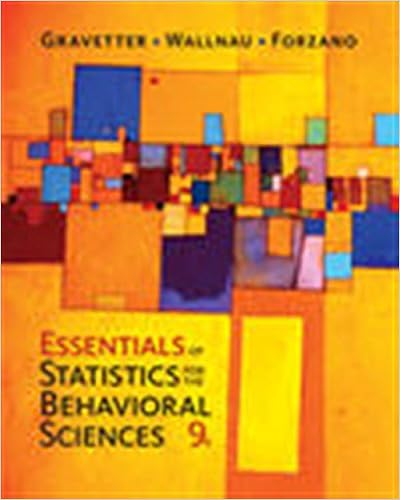# A researcher assigns 21 adolescents to rate the

• Test Prep
• 28
• 89% (46) 41 out of 46 people found this document helpful

This preview shows page 19 - 21 out of 28 pages.

##### We have textbook solutions for you!
The document you are viewing contains questions related to this textbook.The document you are viewing contains questions related to this textbook.
Chapter 12 / Exercise 3
Essentials of Statistics for the Behavioral Sciences
Gravetter/WallnauExpert Verified
98.A researcher assigns 21 adolescents to rate the likability of a person described in a vignette as having one of three personality traits. An equal number of participants are assigned to each group. If obtF= 4.08 for this study, then what was the decision at a .05 level of significance for a one-way between-subjects ANOVA?A)Reject the null hypothesis.B)Retain the null hypothesis.C)There is not enough information to answer this question.
99.A researcher computes the following one-way between-subjects ANOVA table. State thedecision at a .05 level of significance. (Hint: Complete the table first.)Source of VariationSSdfMSFBetween groups324Within groups (error)45Total122
100.In a study with three groups and 13 participants in each group, the sum of squares for the within-groups source of variation is 18. What is the value for the mean square within-groups in this study?
101.When the variability attributed to between-groups is equal to the variability attributed toerror, then the value of the test statistic for a one-way between-subjects ANOVA is,
102.Following a significant one-way between-subjects ANOVA in which k> 2, what is the next appropriate step?A)Summarize the data; no further tests are required.Page 19
##### We have textbook solutions for you!
The document you are viewing contains questions related to this textbook.The document you are viewing contains questions related to this textbook.
Chapter 12 / Exercise 3
Essentials of Statistics for the Behavioral Sciences
Gravetter/WallnauExpert Verified
B)Interpret the data; no further tests are required.C)Conduct post hoc tests.D)both A and B
103.Which of the following is not a post hoc test for a one-way between-subjects ANOVA?
104.Which of the following post hoc tests is associated with the least power to detect an effect?
105.Post hoc tests are computed
•••